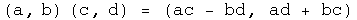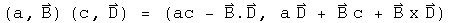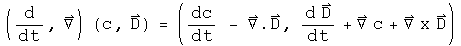Multiplying Quaternions the Easy Way

Multiplying two complex numbers a + b I and c + d I is straightforward.For two quaternions, b I and d I become the 3-vectors B and D, where B = x I + y J + z K and similarly for D. Multiplication of quaternions is like complex numbers, but with the addition of the cross product.Note that the last term, the cross product, would change its sign if the order of multiplication were reversed (unlike all the other terms). That is why quaternions in general do not commute.

If a is the operator d/dt, and B is the del operator, or d/dx I + d/dy J + d/dz K (all partial derivatives), then these operators act on the scalar function c and the 3-vector function D in the following manner:This one quaternion contains the time derivatives of the scalar and 3-vector functions, along with the divergence, the gradient and the curl. Dense notation :-)